### Home > PC > Chapter 4 > Lesson 4.1.3 > Problem4-43

4-43.
1. For each angle (in radians) listed below, determine the signs of the coordinates in a unit circle. Homework Help ✎

1.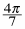2.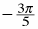3.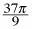4. −21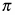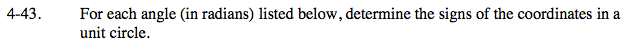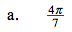1. The denominator says the the top half of the circle should be divided into 7 pieces.

2. The numerator says go to the 4th piece starting from (1, 0)

3. This angle is the 2nd quadrant so the signs are (−, +) for (x, y).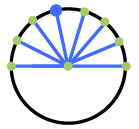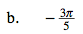Move clockwise from (1, 0).Divide half of the circle into 9 pieces. Every 18 pieces is one time around the circle.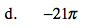−21π intersects the unit circle at the point (−1, 0), so the signs of the coordinates are (−, 0).